#Operations on Fourier SeriesThe results obtained in this page may easily be extended to function defined on any interval [a,b]. So without loss of generality, we will assume that the functions involved are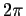-periodic and defined on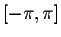.

Let f(x) be a-periodic piecewise continuous function. Then the function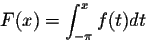is continuous and is-periodic if and only if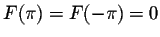, i.e. the Fourier coefficient a0 = 0. It is also quite easy to show that if f(x) is piecewise smooth, then also is F(x). An interesting question will be to find out if a simple relationship between the Fourier coefficients of f(x) and F(x) exist. Denote by An and Bn the Fourier coefficients of F(x). We have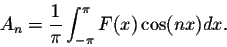Integration by parts will give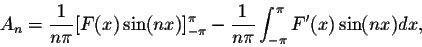for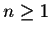. Hence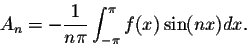A similar calculation gives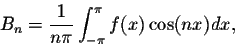and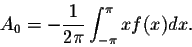This shows the following:

Theorem. Integration of Fourier series
Let f(x) be-periodic piecewise continuous function such that a0 = 0. If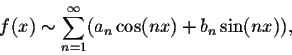then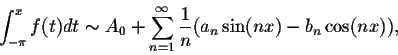where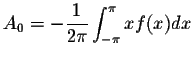.

Since the function F(x) is continuous, we have for any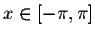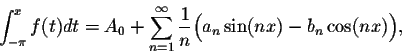because of the main convergence Theorem relative to Fourier series.

Example. Consider the function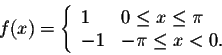We have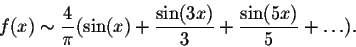Since, for any, we have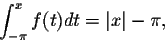then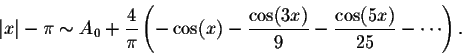Simple calculations give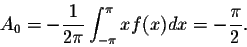Hence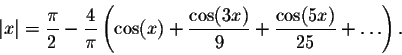Let f(x) be-periodic piecewise continuous function such that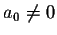. Set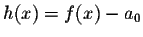. Then h(x) is-periodic piecewise continuous and satisfies the condition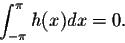Since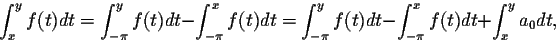the result above implies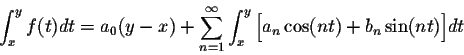which completes the proof.

Theorem. Let f(x) be-periodic piecewise continuous function. Then for any x and y, the integral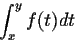may be evaluated by integrating term-by-term the Fourier series of f(x).

Example. In the example above, we showed thatHence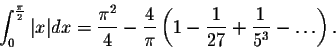This implies the formula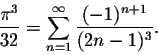This kind of formulas are quite interesting. Indeed, they enable us to find approximations to the irrational number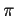.

Example. Show that the trigonometric series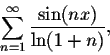is not the Fourier series of any function.
Answer. It is easy to see that this series converges for any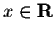. Assume there exists a function f(x) such that this series is its Fourier series. Then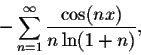must be convergent everywhere since it is going to be the Fourier series of the antiderivative of f(x). But this series fails to be convergent when x=0. Contradiction.

After we discussed the relationship between the Fourier series of a function and its antiderivative, it is natural to ask if a similar relationship exists between a function and its derivative. The answer to this is more complicated. But we do have the following result:

Theoreme. Let f(x) be-periodic continuous and piecewise smooth function. Then, for any, we have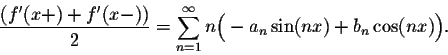In other words, we obtain the Fourier series of f'(x) by differentiating term-by-term the Fourier series of f(x).

Application: Isoperimetric Inequality

Theoreme. Consider a smooth closed curve in the plane xy. Denote by P its perimeter (total arclength) and by A the area of the region enclosed by the curve. Then we have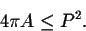The equality holds if and only if the curve is a circle.

Proof. A parametric representation of the curve may be given by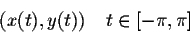with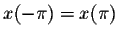and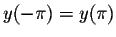. The formulas giving P and A are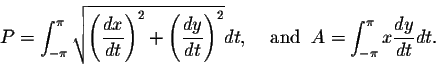Set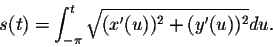Then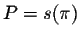. Consider the new variable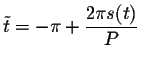. If we rewrite the parametric representation in terms of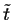, we get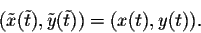Easy calculations give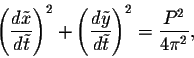i.e. the new variable enables us to reparametrize the curve while assuming the quantity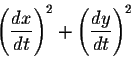constant. Hence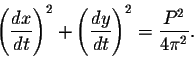Since the curve is smooth, we get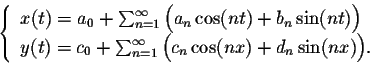Previous result, on the relationship between the Fourier coefficients of the function and its derivative, gives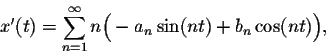and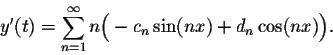Parseval formula implies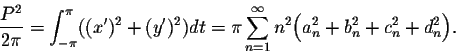On the other hand, we have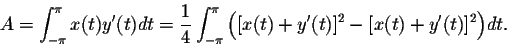Hence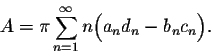Algebraic manipulations imply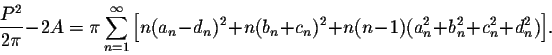Since the second term of this equality is positive, we deduce the first part of the result above. On the other hand, we will have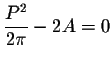if and only if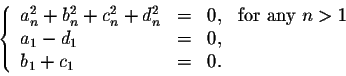This implies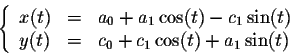for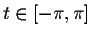. Therefore the curve is a circle centered at (a0,c0) with radius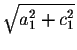, which completes the proof of the theorem.[Geometry] [Algebra] [Trigonometry ]
[Calculus] [Differential Equations] [Matrix Algebra]S.O.S MATH: Home Page

Do you need more help? Please post your question on our S.O.S. Mathematics CyberBoard.Author: M.A. Khamsi